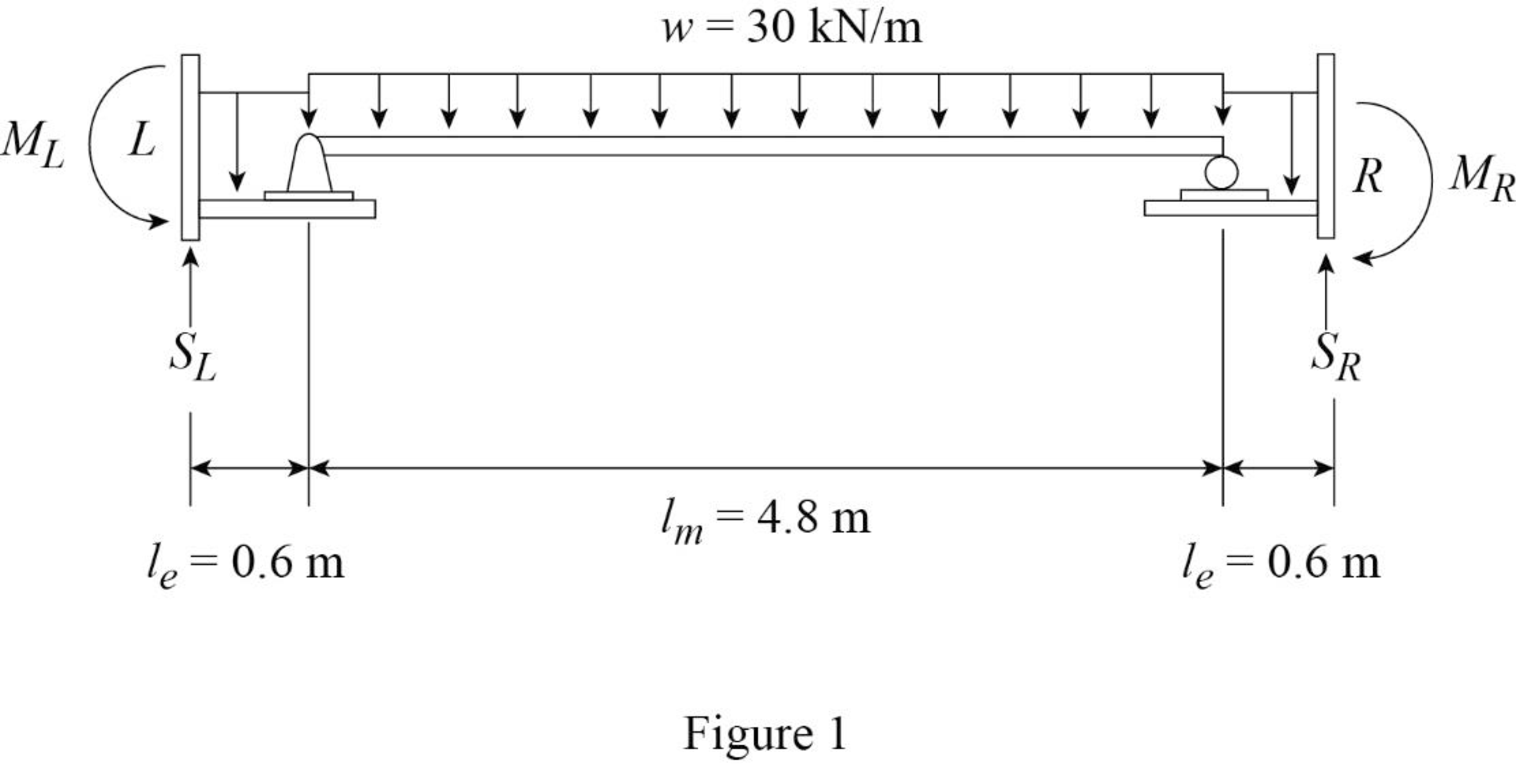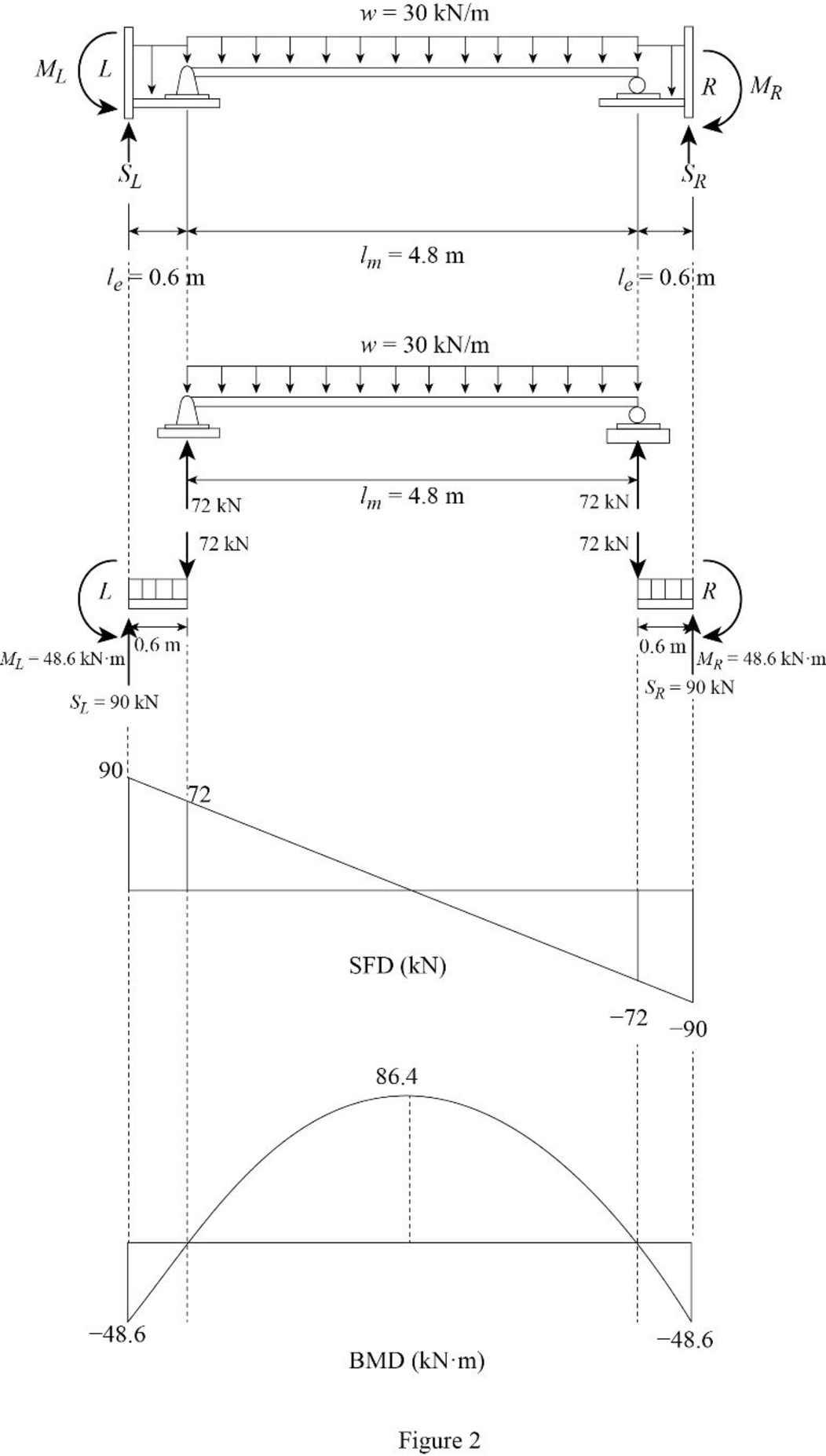# 12.1 through 12.5 Draw the approximate shear and bending moment diagrams for the girders of the frames shown in Figs. P12.1 through P12.5. FIG. P12.1

#### Solutions

Chapter
Section
Chapter 12, Problem 1P
Textbook Problem
768 views

## 12.1 through 12.5 Draw the approximate shear and bending moment diagrams for the girders of the frames shown in Figs. P12.1 through P12.5.FIG. P12.1

To determine

Draw the shear and bending moment diagrams for the girders of given frame.

### Explanation of Solution

Given information:

The uniformly distributed load acting along the girder DEF (w) is 30 kN/m.

The horizontal distance of the point AB and BC (L) is 6 m.

The vertical distance of the members AD, BE, and CF (lv) is 4 m.

Calculation:

The span length and loads for the two girders of the frame DE and EF are same; therefore the approximate shear and bending moment diagrams for the girders will also be the same.

Consider the girder DE.

Determine the span for the middle portion of the girder using the relation.

lm=0.8L

Substitute 6 m for L.

lm=0.8×6=4.8m

Determine the span for the two end portion of the girder using the relation.

le=0.1L

Substitute 6 m for L.

le=0.1×6=0.6m

Draw the statically determinate girder portion as in Figure (1).Consider the equilibrium of the simply supported middle portion of the girder.

Determine the vertical reactions at the end portion using the relation.

V=wlm2

Substitute 30 kN/m for w and 4.8 m for lm.

V=30×4.82=72kN

Consider the equilibrium conditions of the end portions of the girder.

Consider upward direction is positive and counter clockwise moment is positive.

Determine the support reaction at the left end.

Apply the equations of equilibrium to the left end portion.

FY=0SLw×leV=0

Substitute 30 kN/m for w, 0.6 m for le, and 72 kN for V.

SL30×0.672=0SL=90kN()

Determine the moment at the left end.

Take moment about left end is equal to zero.

ML=0MLw×le×le2V×le=0

Substitute 30 kN/m for w, 0.6 m for le, and 72 kN for V.

ML30×0.6×0.6272×0.6=0ML=48.6kNm(Counterclockwise)

Consider upward direction is positive and clockwise moment is positive.

Determine the support reaction at the right end.

Apply the equations of equilibrium to the right end portion.

FY=0SRw×leV=0

Substitute 30 kN/m for w, 0.6 m for le, and 72 kN for V.

SR30×0.672=0SR=90kN()

Determine the moment at the right end.

Take moment about right end is equal to zero.

MR=0MRw×le×le2V×le=0

Substitute 30 kN/m for w, 0.6 m for le, and 72 kN for V.

MR30×0.6×0.6272×0.6=0MR=48.6kNm(Clockwise)

Determine the maximum bending moment at the middle of the girder using the relation.

Mmax=wlm28

Substitute 30 kN/m for w and 4.8 m for lm.

Mmax=30×4.828=86.4kNm

Draw the shear force and bending moment diagram as in Figure (2).#### The Solution to Your Study Problems

Bartleby provides explanations to thousands of textbook problems written by our experts, many with advanced degrees!

Get Started

Find more solutions based on key concepts
VocabularyState the meaning of the following terms: Outcome _____ A Population _____ Frequency Distribution ___...

Engineering Fundamentals: An Introduction to Engineering (MindTap Course List)

What feature of mating threads determines the class of fit?

Precision Machining Technology (MindTap Course List)

Explain how to use a micrometer to measure cam lobe wear.

Automotive Technology: A Systems Approach (MindTap Course List)

Differentiate among the following technology user types: home user, small/home office user, mobile user, power ...

Enhanced Discovering Computers 2017 (Shelly Cashman Series) (MindTap Course List)

If your motherboard supports ECC DDR3 memory, can you substitute non-ECC DDR3 memory?

A+ Guide to Hardware (Standalone Book) (MindTap Course List)

The AWS Visual Inspection Criteria allows one small porosity per inches of weld.

Welding: Principles and Applications (MindTap Course List)